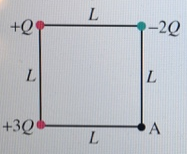# Problem: Three point charges are arranged at the corners of a square of side L as shown in (Figure 1) What is the potential at the fourth corner (point A)? Express your answer in terms of the variables Q, L, and the Coulomb's constant k.

###### FREE Expert Solution

Potential:

$\overline{){\mathbf{V}}{\mathbf{=}}\frac{\mathbf{k}\mathbf{Q}}{\mathbf{d}}}$

At point A, the potential will be:

VA = V-2Q + V+Q + V+3Q

86% (185 ratings)###### Problem Details

Three point charges are arranged at the corners of a square of side L as shown in (Figure 1)What is the potential at the fourth corner (point A)? Express your answer in terms of the variables Q, L, and the Coulomb's constant k.

Frequently Asked Questions

What scientific concept do you need to know in order to solve this problem?

Our tutors have indicated that to solve this problem you will need to apply the Electric Potential concept. You can view video lessons to learn Electric Potential. Or if you need more Electric Potential practice, you can also practice Electric Potential practice problems.

What professor is this problem relevant for?

Based on our data, we think this problem is relevant for Professor Werhner's class at UCF.